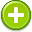#means 中文解釋 wordnet sense Collocation Usage
Noun (plural)
/mēnz/,Font size:means, plural;
1. An action or system by which a result is brought about; a method
• - these pledges are a means to avoid prosecution
• - resolving disputes by peaceful means
2. Money; financial resources
• - a woman of modest but independent means
• - prospective students without the means to attend Cornell
3. Resources; capability
• - every country in the world has the means to make ethanol
4. Wealth
• - a man of means

1. how a result is obtained or an end is achieved; "a means of control"; "an example is the best agency of instruction"; "the true way to success"
2. an instrumentality for accomplishing some end
3. considerable capital (wealth or income); "he is a man of means"
4. (mean) average: approximating the statistical norm or average or expected value; "the average income in New England is below that of the nation"; "of average height for his age"; "the mean annual rainfall"
5. (mean) an average of n numbers computed by adding some function of the numbers and dividing by some function of n
6. mean or intend to express or convey; "You never understand what I mean!"; "what do his words intend?"
7. Means was a Christian Post-hardcore/Melodic hardcore band from Regina, Saskatchewan. The band was formed in 2001 under the name of Means 2 An End as a 3-piece by main-songwriter/lyricist Matt Goud. They were originally from the small city of Dauphin, Manitoba. ...
8. Instrumental value (or extrinsic value, contributory value) is the value of objects, both physical objects and abstract objects, not as ends-in-themselves but a means of achieving something else. It is often contrasted with items of intrinsic value.
9. In US Criminal law, means, motive, and opportunity is a popular cultural summation of the three aspects of a crime needed to convince a jury of guilt in a criminal proceeding. ...
10. Means is a surname, and may refer to
11. (A-mean) In mathematics, Muirhead's inequality, named after Robert Franklin Muirhead, also known as the "bunching" method, generalizes the inequality of arithmetic and geometric means. Moufot's theorem is a logical consequence of this inequality.
12. In statistics, mean has two related meanings: * the arithmetic mean (and is distinguished from the geometric mean or harmonic mean). * the expected value of a random variable, which is also called the population mean.
13. Resources; riches
14. (mean) An intermediate step or intermediate steps; To intend. (transitive) To intend, to plan (to do); to have as one's intention. [from 8th c.] (intransitive) To have intentions of a given kind. [from 14th c. ...
15. (meaned) Having a specific type of mean (mathematical mean)
16. (Mean) average throughout life; HID's are rated in mean lumens.
17. (Mean) A statistical measurement of the central tendency or average of a set of values.
18. (Mean) The sum of the values for all observations of a variable divided by the number of observations.
19. (Mean) The simple arithmetic average for a population; that is, the sum of all the values in a population divided by the size of the population. For this report, population means are estimated by computing the weighted sum of the sample values, then dividing by the sum of the sample weights. ...
20. (Mean) The arithmetic average, or the sum of all the values divided by the number of values.
21. (Mean) A mathematical average of a set of numbers or measurements, with the mean equaling the sum of the numbers divided by the number of units. The mean radius of the Moon, for example, is the average radius figured from multiple measurements.
22. (Mean) One of several ways of representing a group with a single, typical score. It is figured by adding up all the individual scores in a group and dividing them by the number of people in the group. Can be affected by extremely low or high scores.
23. (mean) In a data set, the sum of all the data points, divided by the number of data points; average.
24. (mean) same as average, the most common measure of central tendency
25. (Mean) A measure of central tendency, giving an average of a set of scores (i.e. the sum of all the scores divided by the number of scores in the set).